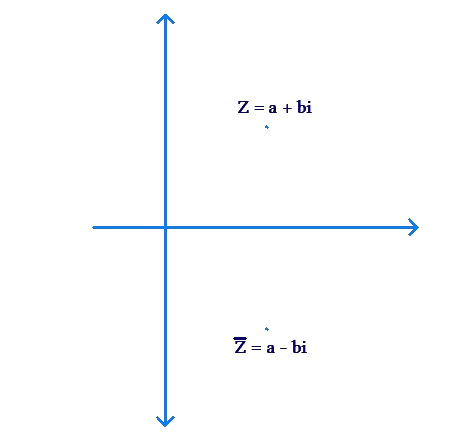# Conjugate of a complex Number

Go back to  'Complex Numbers'

For an arbitrary complex number $$z = a + bi$$, its conjugate is defined as $$\bar z = a - bi$$. For example,

\begin{align} z = 2 + 3i & \Rightarrow \bar z = 2 - 3i \\ z = - 1 - 5i & \Rightarrow \bar z = - 1 + 5i \\ z = i - \frac{1}{2} & \Rightarrow \bar z = - i - \frac{1}{2}\end{align}

Note the last example carefully. When we take the conjugate of a complex number, it is the imaginary part which reverses in sign, and not the real part.

Geometrically, $$\bar z$$ will be the mirror image of z in the horizontal axis, as the following figure shows:We make the following observations:

• The real part of $$\bar z$$ is the same as the real part of z. That is, $${\mathop{\rm Re}\nolimits} \left( {\bar z} \right) = {\mathop{\rm Re}\nolimits} \left( z \right)$$.

• The imaginary part of $$\bar z$$ is the negative of the imaginary part of z. That is, $${\mathop{\rm Im}\nolimits} \left( {\bar z} \right) = - {\mathop{\rm Im}\nolimits} \left( z \right)$$.

• If z is purely real, then $$\bar z = z$$. For example, if $$z = 3$$, then $$\bar z$$ is also 3.

• If z is purely imaginary, then $$\bar z = - z$$. For example, if $$z = 2i$$, then $$\bar z = - 2i$$.

• If z is above the horizontal axis, then $$\bar z$$ is below the horizontal axis, and vice-versa.

Now, let’s see what happens if we add / subtract a complex number and its conjugate. Let $$z = a + bi$$. We have:

\begin{align}&z + \bar z = \left( {a + bi} \right) + \left( {a - bi} \right) = 2a = 2{\mathop{\rm Re}\nolimits} \left( z \right)\\&z - \bar z = \left( {a + bi} \right) - \left( {a - bi} \right) = 2bi = 2i{\mathop{\rm Im}\nolimits} \left( z \right)\end{align}

By virtue of these relations, we can write the real and imaginary parts of a complex number as follows:

\begin{align}&{\mathop{\rm Re}\nolimits} \left( z \right) = \frac{{z + \bar z}}{2}\\&{\mathop{\rm Im}\nolimits} \left( z \right) = \frac{{z - \bar z}}{{2i}}\end{align}

Next, consider what will happen if we multiply z and $$\bar z$$:

\begin{align}z\bar z& = \left( {a + bi} \right)\left( {a - bi} \right)\, = {\left( a \right)^2} - {\left( {bi} \right)^2}\\& = {a^2} - {b^2}{i^2} = {a^2} - {b^2}\left( { - 1} \right)\\& = {a^2} + {b^2} = {\left| z \right|^2}\end{align}

Thus, the product of a complex number and its conjugate gives the square of its magnitude. This relation is used very frequently, and should be internalized properly.

Example 1: Consider the following two complex numbers:

\begin{align}&{z_1} = 2 - 3i\\&{z_2} = - 4 - 7i\end{align}

Find the conjugate of the complex number $$z = 4{z_1} - 2i{z_2}$$.

Solution: We have:

\begin{align}&4{z_1} = 4\left( {2 - 3i} \right) = 8 - 12i\\&2i{z_2} = 2i\left( { - 4 - 7i} \right) = - 8i - 14{i^2} = 14 - 8i\\& \Rightarrow \,\,\,z = 4{z_1} - 2i{z_2} = \left( {8 - 12i} \right) - \left( {14 - 8i} \right)\\ &= - 6 - 4i\end{align}

Thus,

$\bar z = - 6 + 4i$